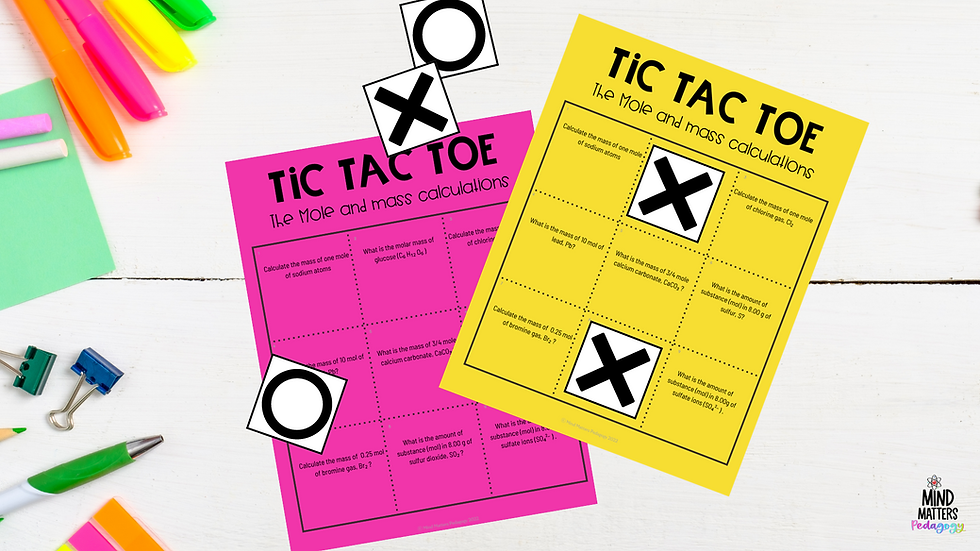top of page
• Androy

# Using Tic-Tac-Toe to Make mole calculations more accessible to chemistry studentsIf you have been following my blog or part of my mailing list then you should know that I absolutely love using game-based learning in my chemistry classroom. Why? because it is less intimidating to students than a worksheet. There is some science behind it that I won't go into in this post, but just know that game-based learning is beneficial for two main reasons:

1. Students forget they’re learning while they’re learning: Sometimes students just need some low-stakes activities to take their mind off the work part and have fun, but they are still learning during the process.

2. The games promote critical thinking and problem-solving as students need to develop different strategies in order to potentially “win” the game and outsmart their opponent.

What better topic to use game-based learning for than the mole concept and mole calculations?

## How I use tic-tac-toe for pair work

I don't know about you, but my students hate the mole, there is just something about all those small numbers that they dislike, and usually, they get lost somewhere and then... it's a whole mess.

Thankfully teaching the mole concept using dimensional analysis was removed from our syllabus because our students are notoriously terrible at math. No matter what teaching strategy you use, students need practice in order to master these calculations and that is why I use tic tac toe as part or pair work in order to get my students to that level.

### How to play

After I have covered the topic of the mole, Avogadro's number and molar mass I place students in pairs. You need to be strategic when pairing students off as this will have a direct impact on how effective the activity will be.

• I pair students of mixed abilities together because my goal is to get them to learn from each other and essentially practice together.

• The game is played in the traditional way, i.e. the first player to get three of their marks in a row (up, down, across, or diagonally) is the winner. When all nine squares are full, the game is over. If no player has three marks in a row, the game is tied.

• With this game, each grid has nine mole calculations of varying difficulty. In order for any student to place a mark (x or O) on a square, they must first solve the problem in that square. The added challenge is that students may have to answer a question they would usually dodge in order to have an advantage in the game.

• Each student pair is given a blank worksheet on which they will work out the problem and provide an answer to the question on the grid. This is a way for me to check that students have done the work and I also use this as a formative assessment.

• To keep students accountable I encourage both team members to work on the problem on the grid and to compare their answers. I make it explicit at the beginning of the activity that although you want to "win" the primary focus of the activity is to practice these problems. Discrepancies in student answers leave room for discussion.

### Make it a challenge

Sometimes I challenge students by adding a timed component to the game, i.e. each student is only allowed 3 min to answer the question, but that is only done towards the end of the term when I'm sure students are comfortable with the calculations.

## Three Categories of Worksheets

Instead of bombarding students with mixed mole problems off the bat, each tic-tac-toe grid is focused on one type of calculation.

### 1. Moles and Mass Calculations

This grid focuses on calculating molar mass, converting a given number of moles to mass and converting a given mass to moles. I also focus on using the correct terminology for the different particles here for example:

What is the mass of 3/4 mole of oxygen gas and make a distinction what is the mass of 3/4 mole of oxygen atoms?

This is important so that students understand the difference between atoms, molecules, and formula units, ions. I have noticed that confusion of these terms often leads to incorrect calculations.

### 2. Moles and Number of particles

This grid focuses on using Avogadro's number to calculate the number of particles given the number of moles and vice versa.

I have found students do not like dealing with numbers written in scientific notation. I usually give them a bit of practice with as well as rounding and calculations with significant figures by giving them these quick assessment worksheets for homework.

### 3. Moles, Mass and Number of particles

The final grid focuses on mixed problems. Students will be required to go from moles to number of particles, mass to number of particles using Avogadro's number and vice versa. This is the most challenging of the three and I only allow students to complete this when I am sure they are comfortable with the problems in the previous two.

Using these worksheets as opposed to the traditional worksheet approach takes the boring out of practising mole calculations. your students will love it!

bottom of page